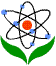Asia-Pacific Forum on Science Learning and Teaching, Volume 5, Issue 3, Article 7 (Dec., 2004) MAN Yiu Kwong Reflection: its concepts and applications in geometry

Some applications in geometry

We now illustrate how to apply the principle of reflection in geometry in the following examples.

Example 1 (The Cowboy Problem)
In Figure 4, a cowboy is located at C. Before riding on his horse to the campsite S, he wants to ride his horse to get some grass at the edge of the field and then go to the river for a drink. What is the shortest path from C to the edge of the grass field, to the river and then to S?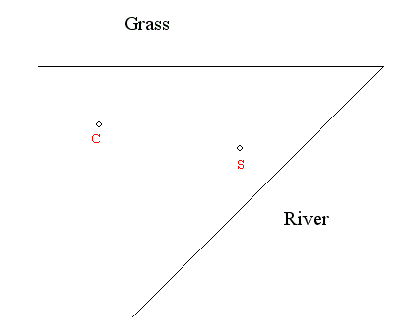Figure 4

To start off, we have to look for the positions of the points P and Q, where the cowboy stops at the edge of the field and at the river, respectively. How can we locate these two points? First, we find the mirror images C' and S' by reflections. Next, we join C' and S' to obtain a line segment. The intersections between this line segment and the edges of the field and the river will fix the positions of P and Q. Thus, the shortest path required is CPQS, as shown in Figure 5.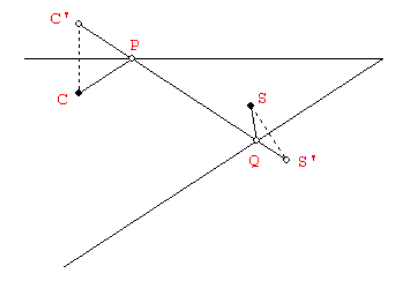Figure 5

To prove its correctness, we first note that CP + PQ + QS = C'P + PQ + QS', which means the total length of the path is equal to the length of C'S'. Now, suppose the cowboy stops at other arbitrary points on the edges, say P' and Q'. By reflections, the total distance traveled will be equal to C'P' + P'Q' + Q'S', which is greater than C'S' because C'P'Q'S' is not a straight line, as illustrated in Figure 6. We can see that the built-in measurement tool of dynamic geometry software is quite convenient for illustration or self-exploration of the concepts involved.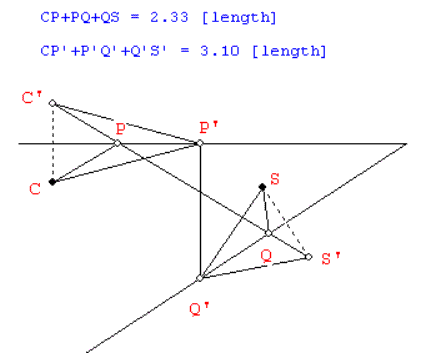Figure 6

Example 2 (The Billiard Ball Problem)

In Figure 7, a billiard ball is placed at a point D in a triangular billiard table ABC. If the ball is projected to the side AB, and rebounds to BC and then to CA, what is the path of the ball if it can return to D after the three rebounds? Assume there is no energy lost when the ball hits the sides of the table.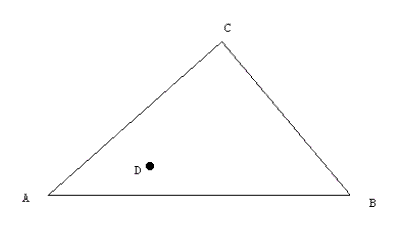Figure 7

First, we have to look for the positions of the points P, Q and R, where the ball rebounds from AB, BC and CA, respectively. Applying reflections is a good start, so we find the mirror images D1 and D2 by reflections, with respect to AB and CA, respectively. Although we do not know where are the locations of P, Q and R yet, we can get some ideas by adding an arbitrary point Q' on BC and then draw the line segments Q'D1 and Q'D2, as shown in Figure 8. It is easy to see that the angle of incidence is equal to the angle of reflection at either E or F because DEA =Q'EC and DFA =Q'FB. However, EQ'C may not be equal to FQ'B. Again, a dynamic geometry software is a good tool for us to explore the exact position of Q by dragging Q' along BC and allow us to observe the measures of EQ'C and FQ'B.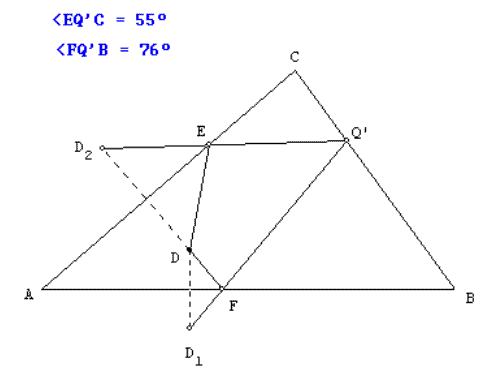Figure 8

Figure 9 shows the desired position of Q' and so we can replace the labels F, Q' and E by P, Q and R, respectively, to obtain the path of the ball in Figure 10.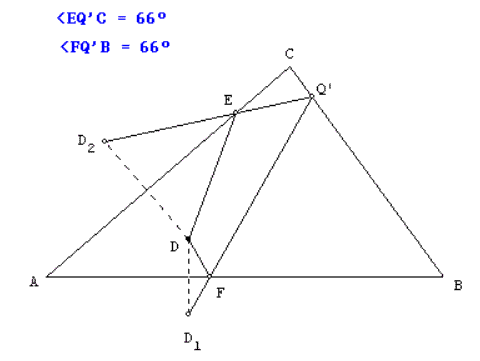Figure 9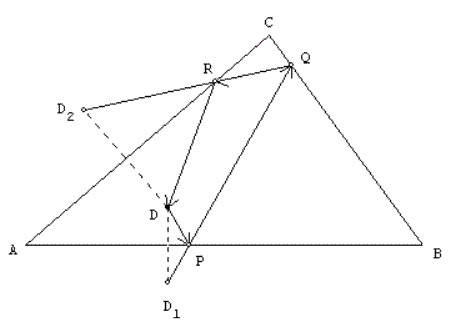Figure 10

A further exploration by dynamic geometry software also reveals that the mirror image of D1 with respect to BC, say D3, must lie on the extension of D2Q. It is because PQB = D3QB = RQC, and so D2, Q and D3 are collinear, as shown in Figure 11. Hence, we know that a quick solution to this billiard ball problem is to find the mirror images D1, D2 and D3 first, and draw the line segment D2D3 to locate the positions of R and Q, and then draw the line segment D1Q to locate the position of P. Joining the points D, P, Q and R will give us the path required.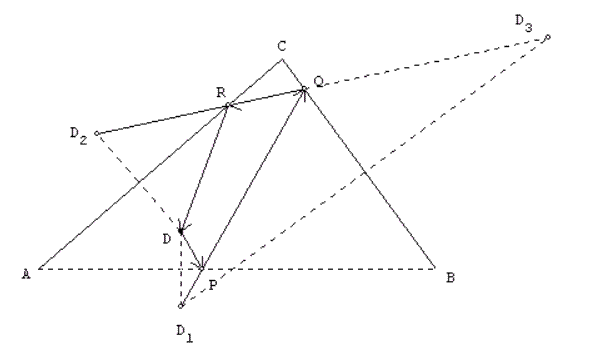Figure 11

In fact, we can further deduce that the ball can return to its original position provided the line segment D2D3 intersects with the line segments BC and CA. However, we have to point out that this example is a slight deviation to the real-life situation in which the energy lost is normally inevitable and the angle of incidence need not be equal to the angle of reflection in such a case.